Graph Gallery, page 1The descriptions list the function/s which produced the graph and the range of the variable/s used.
Click a thumb-nail graphic for an enlarged version.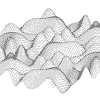z = Cos(x+2*pi*cos(y/2))+Cos(y+2*pi*Cos(x/2))x = -9 to 9, y = -9 to 9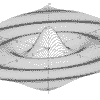z = (x*x-y*y)^2/(x*x+y*y) x = -9 to 9, y = -9 to 9 Psuedo-Bessell function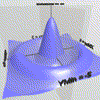Surface plot of z = 4*(x*x-y*y)^2/(x*x+y*y) x = -5 to 5, y = -5 to 5 Psuedo-Bessell function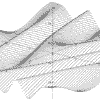z = Cos(x+2*pi*Cos(y/2)) x = -2pi to 2pi, y = -2pi to 2pi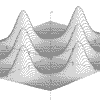z = Exp(Sin(x)*Sin(y)*3)/4 x = -2pi to 2pi, y = -2pi to 2pi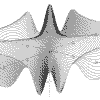z = Cos(x*x-y*y) x = -pi to pi, y = -pi to pi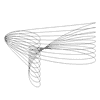x = 2*Cos(t) + Sin(2*t) y = Sin(t) + Cos(2*t) z = t*Cos(t)/10 t = -30 to 30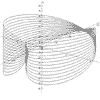x = 2*Cos(t) + Cos(2*t) y = 2*Sin(t) + Sin(2*t) z = t*Sin(t) t = -50 to 50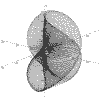r = Sqr(4*Cos(a + b)) a = 0 to 2pi, b = 0 to 2pi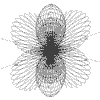r = Sin(2*a) + Sin(2*b) a = 0 to 2pi, b = 0 to 2pi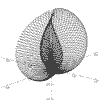r = Sin(a) + Sin(b) a = -pi to pi, b = -pi to pi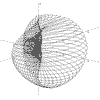r = 1/Sqr(a*a + b*b) a = 0 to 2pi, b = 0 to 2pi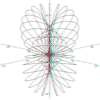Red/blue anaglyph r = sin(a + b) - cos(a + b) a = 0 to 2pi b = 0 to 2pi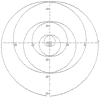x=cos(t) + t^2*sin(t) y=sin(t) + t^2*cos(t) t = -8pi to 8pi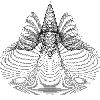z = e^(-r*r)*(sin(2*r)-r*cos(4*a)) r = 0 to 2 a = -2pi to 2pi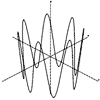r =2 a = t z =cos(7*t) t = -pi to pi